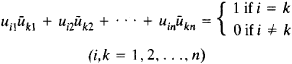# Unitary Matrix

(redirected from Special unitary matrix)
Also found in: Dictionary.

## unitary matrix

[′yü·nə‚ter·ē ′mā·triks]
(mathematics)
A matrix whose inverse is equal to the complex conjugate of its transpose.

## Unitary Matrix

A unitary matrix of order n is an n × n matrix [uik] with complex entries such that the product of [uik] and its conjugate transpose [ūki] is the identity matrix E. The elements of a unitary matrix satisfy the relationsThe unitary matrices of order n form a group under multiplication. A unitary matrix with real entries is an orthogonal matrix.

Site: Follow: Share:
Open / Close